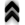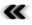### 4.2 Algebraic Structures

Beside the bare structures described so far, the structures resulting from operations deﬁned on top of the sets as a combined union gives rise to algebraic structures. They are of importance as they provide rules, how elements interacts and create new elements.

In the setting of digital computers types are models or extensions of algebraic structures. Therefore, several algebraic structures are introduced in the following with an increasing number of requirements imposed on elements and/or operations.

Deﬁnition 10 (Monoid) A set of elements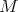on which a binary operation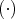is deﬁned is called a monoid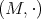, if the binary operation satisﬁes the following conditions:

• Closed: The result of the binary operation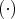is again an element of the initial set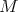: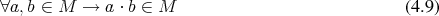• Associativity: The order in which consecutive operations are applied does not change the result: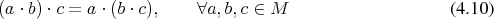• An identity (neutral) element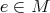exists. such that: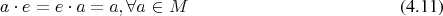Further requirements on Deﬁnition 10 result in

Deﬁnition 11 (Group) A monoid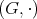, where the binary operation additionally fulﬁls the condition that for every element in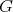an inverse element exists, which produces the identity element under the binary operation is called a group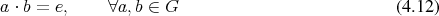and as a further qualiﬁcation

Deﬁnition 12 (Abelian group) When the order of the operands of the binary operation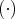of a group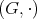does not change its result, the group is called Abelian or commutative.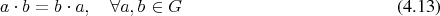Groups are basic building blocks in the exploration of further structures, which can be deﬁned by demanding additional operations on the basic sets.

Deﬁnition 13 (Ring) An Abelian group (Deﬁnition 12), which is equipped with an additional binary operation under which it is a monoid (Deﬁnition 10), is called a ring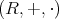, if the two binary relations are distributive: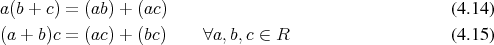The structure of the described ring is, however, insuﬃcient to describe the basic notion of real numbers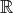or the complex numbers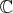. To this end the following deﬁnition is required:

Deﬁnition 14 (Field) If the multiplication operation of a ring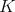is invertible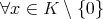, it is called a ﬁeld.

The next deﬁnition describes the algebraic structure of entities (Deﬁnition 61), which have proven to be immensely useful.

Deﬁnition 15 (Module) A Module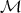over a ring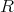(Deﬁnition 13) is an Abelian group (Deﬁnition 12) with respect to the operation of the addition of two elements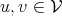, while additionally being a ring with respect to the operation of multiplying elements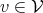by elements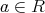, which are called scalars.

A related deﬁnition with somewhat stronger requirements yields a structure, which is essential for the construction of simple geometric settings.

Deﬁnition 16 (Vector space) A vector space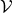over a ﬁeld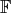is an Abelian group with respect to the operation of the addition of two elements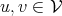, while additionally being a ring with respect to the operation of multiplying elementsby elements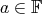. Elements of a vector space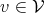are called vectors.

While the elements of vector spaces, the vectors, constitute a powerful concept, they are insuﬃcient to describe all the entities required in modelling scientiﬁc processes. Additional entities are therefore required. It is not limited to entities introduced later and therefore provided here to clearly distinguish the algebraic structure from the elements.

Deﬁnition 17 (Algebra) An algebra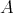over a ﬁeld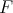is a vector space equipped with an additional binary relation (Deﬁnition 4)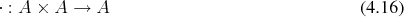A further qualiﬁcation of the just deﬁned structure may be possible. The availability of the following term allows a more precise classiﬁcation as found in Deﬁnition 56 and in conjunction with Deﬁnition 60.

Deﬁnition 18 (Graded algebra) In case the algebra admits the decomposition into additive groups of the form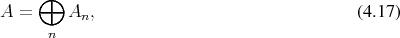where additionally the multiplication operation results in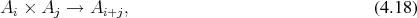it is called a graded algebra.

Among the most versatile and useful, almost ubiquitous, algebraic entities are:

Deﬁnition 19 (Polynomials) A formal prescription of the form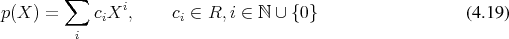with coeﬃcients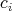is called a polynomial over the ring (Deﬁnition 13)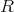in the variable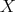.

The variable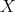in the purely algebraic deﬁnition is a formal symbol and need not be an element of a ﬁeld (Deﬁnition 14), such as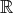or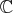, as in the case of polynomial codes. The algebraic considerations, however, assert that polynomials deﬁned in this fashion can be added (subtracted) and multiplied, thus forming a ring (Deﬁnition 13). The case that the variables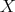are either from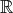or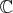is of particular usefulness in many ﬁelds of mathematics, with the ﬁeld of interpolation as well integration among them. Then the expression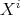is simply theth power of a variable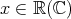and values can be derived by simple multiplication within their respective ﬁelds.